﻿ 电能质量信号重构的广义–正则化正交匹配追踪算法

# 电能质量信号重构的广义–正则化正交匹配追踪算法A Generalized-Regularized Orthogonal Matching Pursuit Algorithm for Power Quality Signal Reconstruction

Abstract: A generalized-regularized orthogonal matching pursuit (GROMP) algorithm is proposed based on compressed sensing theory, and it is used for power quality signal reconstruction. Firstly, the GROMP algorithm selects atoms based on the generalized orthogonal matching pursuit algorithm to form the original support set. Then, the regularization method is added to select atoms from original support set to form the final support set. At last, the least square method is employed to update the residual and reconstruct the original signal. The proposed GROMP algorithm not only compensates for the low accuracy of the generalized orthogonal matching pursuit algorithm, but also overcomes the disadvantages of the poor stability and large computational complexity of the regularized orthogonal matching pursuit algorithm. The simulation results of transient and steady-state power quality signal reconstruction show that the newly proposed GROMP algorithm has good adaptability to a variety of measurement matrices. Compared with the traditional gener-alized orthogonal matching pursuit algorithm and the regularized orthogonal matching pursuit algorithm, the new GROMP algorithm has high reconstruction accuracy for various power quality signals and good stability under small compression ratio.

1. 引言

2. 压缩采样和信号重构

2.1. 压缩采样和信号重构的原理

$y=\Phi x=\Phi \Psi \alpha =\Theta \alpha$ (1)

$\mathrm{min}{‖{\Psi }^{\text{T}}x‖}_{0}\text{ }\text{\hspace{0.17em}}\text{s}\text{.t}\text{.}\text{\hspace{0.17em}}\text{\hspace{0.17em}}y=\Theta \alpha =\Phi \Psi \alpha$ (2)

2.2. 稀疏基和测量矩阵的选择

3. 广义–正则化正交匹配追踪算法

3.1. 广义正交匹配与正则化正交匹配追踪算法

GOMP算法首先给定初始残差(如可将观测信号 $y$ 视为初始残差)，然后每次选取传感矩阵 $\Theta$ 中与残差内积最大的S个原子作为支撑集，并通过最小二乘法更新残差。选取原子时每次只选择与残差相关最大的 $S\left(S 个。

ROMP则是在选取原子时每次选取与残差最相关的K列，再通过正则化的能量筛选选出传感矩阵 $\Theta$ 中满足要求的列作为支撑集，再通过最小二乘更新残差。虽然选取K列提高了精度，但同时增加了算法的复杂度与运行时间。

3.2. 广义–正则化正交匹配追踪算法

1) 初始化：测量矩阵 $\Phi$ ，稀疏基 $\Psi$ ，初始残差 ${r}_{0}=y$ ( $y$ 为观测信号)，循环次数k，传感矩阵 $\Theta$ ，索引集合 ${A}_{k}$ 存储第k次循环中传感矩阵 $\Theta$ 被选择的列序号， ${A}_{0}=\varnothing$${C}_{k}$ 表示第k次循环中依照索引 ${A}_{k}$$\Theta$ 中选取的列集合。

2) 相关性选择：计算相关性 $u=|{\Theta }^{\text{T}}{r}_{k-1}|$ ，从中选取最大的S个值，对应矩阵 $\Theta$ 的列序号构成原始支撑集 $J$

3) 正则化选择：按照正则化原理在 $J$ 中选择最终支撑集 ${J}_{0}$ ，即 $\forall i,j\in {J}_{0}$ 满足 $|u\left(i\right)|\le 2|u\left(j\right)|$ 且能量 ${\sum |u|}^{2}$ 最大。

4) 合并选择：令 ${A}_{k}={A}_{k-1}\cup {J}_{0}$ 来更新存储列序号的集合，对于所有 $j\in {J}_{0}$${C}_{k}={C}_{k-1}\cap {\Theta }_{j}$ 来更新选择的列集合(其中 ${\Theta }_{j}$ 表示 $\Theta$ 的j列)。

5) 更新残差：利用 $y={C}_{k}{\theta }_{k}$ ，通过最小二乘法得到初步估计 ${\theta }_{k}={\left({C}_{k}^{\text{T}}{C}_{k}\right)}^{-1}{C}_{k}^{\text{T}}y$ ，并以此更新残差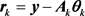6)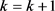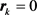则跳出循环，否则返回步骤2)。

7) 最终的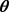即为所求的4. 能质量信号重构的仿真与分析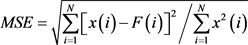(3)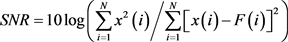(4)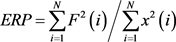(5)Table 1. Seven kinds of power quality signals

4.1. 测量矩阵的适应度仿真Figure 1. Reconstruction of harmonic signal based on three measurement matrices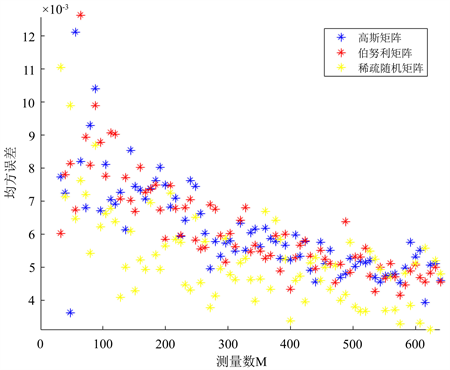Figure 2. Matrix error comparison of harmonic signal based on three measurement matrices

4.2. 电能质量信号重构与误差分析

4.2.1. 稳态信号重构误差分析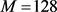的情况下对表1中谐波与间谐波(均值为0的高斯白噪声)两种稳态信号进行重构仿真，结果如图3图4给出了本文提出的算法与GOMP，ROMP关于谐波重构的均方误差MSE，信噪比SNR与能量恢复系数ERP的对比，图5给出这三种算法间谐波进行重构后的三种性能指标的对比，结果如下：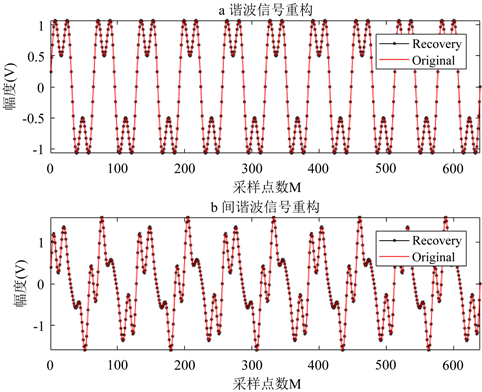Figure 3. Reconstruction of two steady state signals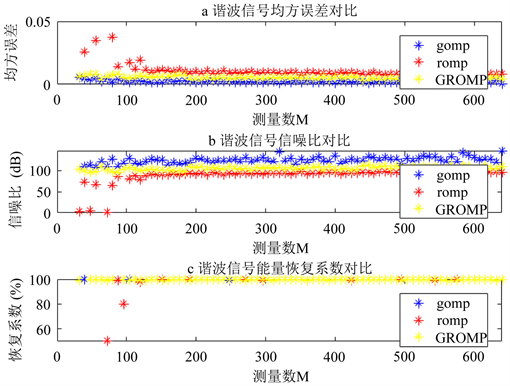Figure 4. Three performance index comparison of harmonic signalsFigure 5. Three performance index comparison of inter-harmonic signalsTable 2. Performance index of three kinds steady state power quality signals

4.2.2. 暂态信号重构仿真与误差分析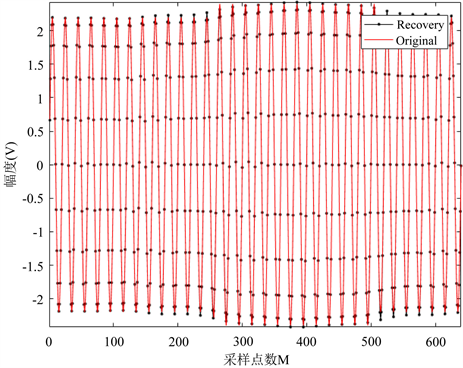Figure 6. Reconstruction of voltage bump signal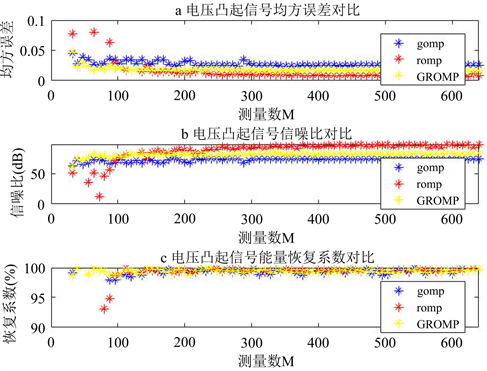Figure 7. Three performance index comparison of voltage bump signal

4.2.3. 复杂信号重构与误差分析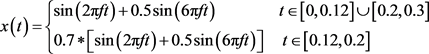Table 3. Performance index of four kinds transient power quality signals

4.3. 三种算法的用时比较Figure 8. Reconstruction of mixed signal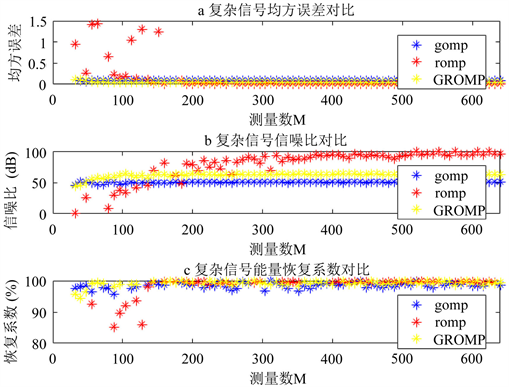Figure 9. Three performance index comparison of mixed signal

5. 结论

 Khan, I., Xu, Y.L., Kar, S. and Sun, H.B. (2017) Electrical and Compressive Sensing-Based Optimal Reactive Power Control of a Multi-Area Power System. IEEE Access, 5, 23576-23588.
https://doi.org/10.1109/ACCESS.2017.2752178

 李天云, 赵妍, 李楠, 冯国, 高宏慧. 基于HHT的电能质量检测新方法[J]. 中国电机工程学报, 2005, 25(17): 52-56.

 Gerek, N. and Ece, D.G. (2004) 2-D Analysis and Compression of Power Quality Event Data. I-EEE Transactions on Power Delivery, 19, 791-798.
https://doi.org/10.1109/TPWRD.2003.823197

 Donoho, D.L. (2006) Compressed Sensing. IEEE Transactions on In-formation Theory, 52, 1289-1306.
https://doi.org/10.1109/TIT.2006.871582

 陈雷, 郑德忠, 廖文喆. 基于压缩感知的含扰动电能质量信号压缩重构方法[J]. 电工技术学报, 2016, 31(8): 163-171.

 Tropp, J.A. and Gilbert, A.C. (2007) Signal Recovery From Random Measurements Via Orthogonal Matching Pursuit. IEEE Transactions on Information Theory, 53, 1-17.

 Needell, D. and Tropp, J.A. (2009) CoSaMP: Iterative Signal Recovery from Incomplete and Inaccurate Samples. Applied and Computation Harmonic Analysis, 26, 301-321.
https://doi.org/10.1016/j.acha.2008.07.002

 Donoho, D.L., Tsaig, Y. and Drori, S.J.L. (2012) Sparse Solution of Under-Determined Linear Equations by Stage Wise Orthogonal Matching Pursuit. IEEE Transactions on Information Theory, 58, 1094-1121.
https://doi.org/10.1109/TIT.2011.2173241

 Do, T.T., Gan, L., Nguyen, N. and Tran, T.D. (2008) Sparsity Adaptive Matching Pursuit Algorithm for Practical Compressed Sensing. Signals, Systems, and Computers, 10, 581-587.

 Gilbert, A.C., Strauss, M.J. and Tropp, J.A. (2006) Algorithmic Linear Dimension Reduction in the l-1 Norm for Sparse Vectors.
https://arxiv.org/pdf/cs/0608079.pdf

 杨挺, 徐明玉, 袁博. 基于压缩感知的微网谐波分析方法[J]. 天津大学学报, 2016, 49(5): 480-484.

 刘嫣, 汤伟, 刘宝泉. 基于压缩感知的电能质量扰动数据稀疏[J]. 电工技术学报, 2019, 33(15): 3461-3470.

 Wang, J., Kwon, S. and Shim, B. (2012) Generalized Orthogonal Matching Pursuit. IEEE Transactions on Signal Processing, 60, 6202-6216.
https://doi.org/10.1109/TSP.2012.2218810

 Needell, D. and Vershynin, R. (2010) Signal Re-covery from Incomplete and Inaccurate Measurements via Regularized Orthogonal Matching Pursuit. Selected Topics in Signal Pro-cessing, 4, 310-316.
https://doi.org/10.1109/JSTSP.2010.2042412

 李树涛, 魏丹. 压缩传综述[J]. 自动化学报, 2009, 35(11): 1369-1377.

Top